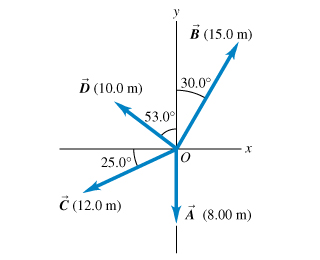# Problem: For the vectors, A, B, and C, in the figure (Figure 1), find the scalar products.A • B =B • C = A • C =

###### FREE Expert Solution

Dot product:

$\overline{)\stackrel{\mathbf{⇀}}{\mathbf{A}}{\mathbf{·}}\stackrel{\mathbf{⇀}}{\mathbf{B}}{\mathbf{=}}{\mathbf{A}}{\mathbf{B}}{\mathbf{c}}{\mathbf{o}}{\mathbf{s}}{\mathbf{\theta }}}$

91% (443 ratings)###### Problem Details

For the vectors, A, B, and C, in the figure (Figure 1), find the scalar products.A • B =

B • C =

A • C =